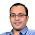Nov 3, 2011

Scaling a Square

Source: Saurabh Joshi, IIT Kanpur

Problem: On a table you have a square made of 4 coins at the corner at distance 1. So, the square is of size 1×1. In a valid move, you can choose any two coin let’s call them mirror and jumper. Now, you move the jumper in a new position which is its mirror image with respect to mirror. That is, imagine that mirror is a centre of a circle and the jumper is on the periphery. You move the jumper to a diagonally opposite point on that circle. With any number of valid moves, can you form a square of size 2×2? If yes, how? If no, why not?

Update (November 4, 2011)

1.nice problem :)

We observe that the parity of the L1 distance between any two coins is an invariant. In 1×1, for any coin, the L1 distance to its neighbors is odd. While in 2×2 every L1 distance between coins is even. Hence not possible

2.No.
If I can go from a 1x1 square to a 2x2 square, then by applying reverse operations, I can also go from a 2x2 square to a 1x1 square.
By applying scaling this means we can also go from a 1x1 square to a 0.5x0.5 square. But this is impossible since if initially all coins are on lattice points, then they remain on lattice points forever. So this leads to a contradiction.

3.brilliant argument!

4.Correct solutions by both sid and Rudradev Basak. Thanks. Saurabh Joshi (whose blog this problem is taken from) gave Rudra's solution and I could also think of that only. Sid's solution seems fresh :)

5.On second thoughts, Rudradev's solution (i.e. Saurabh's solution) is more general. It even works if I am asked to make a 3x3 square. While parity argument is just for even x even squares.

6.So this also extends to any r by r initial square, r \in R? Then there must a plain geometry proof other than Rudradev's?

Fraction Brainteaser

Source: Sent to me by Gaurav Sinha Problem: Siddhant writes a Maths test and correctly answers 5 out of 6 Arithmetic questions and 20...